# BacTesWesley .pdf

### File information

This PDF 1.4 document has been generated by LaTeX with hyperref package / dvips + MiKTeX GPL Ghostscript 8.60, and has been sent on pdf-archive.com on 28/02/2014 at 17:36, from IP address 195.169.x.x. The current document download page has been viewed 809 times.
File size: 227.95 KB (25 pages).
Privacy: public file

### Document preview

Curve25519 in 18 tweets
Bachelor’s Thesis
Author: Wesley Janssen
Supervisor: Peter Schwabe
February 21, 2014

Contents
1 Introduction

2

2 Background and the Problem
2.1 Mathematical background . . . . . . . . . .
2.1.1 Finite Fields . . . . . . . . . . . . .
2.1.2 Elliptic curves . . . . . . . . . . . .
2.1.3 Elliptic curves over Fp . . . . . . . .
2.1.4 Curve25519 . . . . . . . . . . . . . .
2.2 Cryptographic background . . . . . . . . . .
2.2.1 Introduction . . . . . . . . . . . . .
2.2.2 Diffie-Hellman . . . . . . . . . . . .
2.2.3 Elliptic curve Diffie-Hellman . . . .
2.2.4 Timing attacks and countermeasures
2.2.5 NaCl . . . . . . . . . . . . . . . . . .
2.2.6 Previous implementations . . . . . .

.
.
.
.
.
.
.
.
.
.
.
.

.
.
.
.
.
.
.
.
.
.
.
.

.
.
.
.
.
.
.
.
.
.
.
.

.
.
.
.
.
.
.
.
.
.
.
.

.
.
.
.
.
.
.
.
.
.
.
.

.
.
.
.
.
.
.
.
.
.
.
.

.
.
.
.
.
.
.
.
.
.
.
.

.
.
.
.
.
.
.
.
.
.
.
.

3
3
3
4
6
7
8
8
8
9
9
10
11

3 Implementation Details
3.1 Representation of elements of F2255 −19
3.2 Field arithmetic . . . . . . . . . . . . .
3.3 Elliptic curve arithmetic . . . . . . . .
3.4 Packing and unpacking . . . . . . . . .

.
.
.
.

.
.
.
.

.
.
.
.

.
.
.
.

.
.
.
.

.
.
.
.

.
.
.
.

.
.
.
.

12
12
12
15
17

.
.
.
.

.
.
.
.

.
.
.
.

4 Results

18

A Program, seperated in tweets

21

B Program, no layout short version

23

C Program, shortest version

24

1

1

Introduction

There is an average of 1-10 bugs per thousand lines of code in software
of industrial standards [Cov][McC04]. That means that for a project
with 20 million lines of code, there is an average of 20.000 to 200.000
bugs. If this project happens to be a security protocol, bugs can easily
be (maliciously) exploited. An example is in [BBPV12] where a bug in
a function of the OpenSSL implementation is found. Considering that
money transfers, for example, rely on these protocols, where there are
supposedly no bugs, we want to have our ‘secure’ protocols truly secure.
That is why this thesis’s aims is to shrink the code size of an already
existing cryptographic protocol. Specifically, there was a challenge proposed by cryptographer Matthew Green to fit an entire cryptographic library in 100 tweets, in C (1 tweet equals a maximum of 140 characters, so
14.000 characters in total). TweetNaCl is currently the only serious contender in this category. TweetNaCl implements the NaCl library (found
at 2.2.5) in the smallest, still human-readable, form. Its paper can be
found at http://tweetnacl.cr.yp.to/index.html and the entire 100
tweets here: https://twitter.com/TweetNaCl. This bachelor’s thesis is
a part of this paper, and implements one of the five ‘core functions’ of the
NaCl library, namely the key-agreement protocol. NaCl uses curve25519
in its reference implementation, which is introduced here [Ber06]. This
is used as a basis for my own adaptation of this protocol. The five core
functions of NaCl were estimated to take up roughly the same amount
of code, so the goal for this thesis is 100/5 = 20 tweets! This is equal to
20 ∗ 140 = 2800 characters of code.
There have been other attempts to combine twitter’s short-message
constraints and cryptography, as seen for example in [Cyb] and [Twi].
These however, are no serious attempts at making a full cryptographic
library and as such, do not provide the security TweetNaCl (and therefore
this thesis’ algorithm) give.
One of the main focusses is readability. Because there are only a
handful of lines, one can easily check and verify every line in the entire
protocol. To make it easier to read, some conciseness of code has to
be sacrificed, while still being beneath the 20-tweet mark. Another goal
is portability, meaning the implementation is processor-indepedent, and
can be compiled and used on any computer.
In section 2 I explain some of the background information of keyagreement, as well as the mathematics behind it. In section 3 I talk
about the actual implementation. I justify some of the choices I have
made in order to get the code as small as possible, without sacrificing
security or any of the aforementioned goals. In section 4 I briefly present
and discuss the achieved results.

2

2

Background and the Problem

2.1
2.1.1

Mathematical background
Finite Fields

Elliptic curves in cryptography have first been proposed by [Mil86] and
[Kob87]. To understand an elliptic curve defined over a finite field, we
first need to get down to the basics of finite fields, fields in general, and
groups.
A group is a set of elements, together with an operator, which combines any two elements of the group to form a third element. This operator has to satisfy four conditions, also known as the group axioms:
• Closure: all formed elements are also in the group.
• Associativity: ∀a, b, c: (a ◦ b) ◦ c = a ◦ (b ◦ c), where ◦ is the operator
used.
• Identity: there is an element Id, such that ∀a: (a ◦ Id = a).
• Invertibility: every element a in the group has an inverse, such that
a ◦ a−1 = Id.
If, in addition to these, it satisfies the commutativity equation a◦b = b◦a,
it is called an abelian group. In the case of the set of integers combined
with the + operator, ◦ = +, Id = 0, and a−1 = −a, because a + b = b + a,
this is also an abelian group.
A finite field Fp , also called a Galois Field, used in elliptic curve arithmetic, is a field with a finite number of elements in it. The order p of
the field is the number of elements in it. A field is an abelian group with
all conditions from abelian groups. Multiplication is closed, associative
and commutative in the same way addition is. Additionally, it has a
neutral element of its own, 1, where 1 · a = a · 1 = a for some element
a. Every element, except for 0, has an inverse for multiplication as well,
such that a · a−1 = a−1 · a = 1. Lastly, multiplication is distributive with
respect to addition, i.e. ∀a, b, c: (a · (b + c) = a · b + a · c).

3

2.1.2

Elliptic curves

Let F be a field, and E a curve defined over this F. E(F) is the set of
solutions to the following general equation:
y 2 = x3 + ax2 + bx + c
Where a, b, c ∈ F.
For us, Montgomery curves are more interesting because Montgomery
curves are used in the NaCl-library. They generally use less code and are
more resistant to timing attacks than normal elliptic curves [OKS00]. A
Montgomery curve E is of the form:
E(Fp ) = {∞} ∪ {(x, y) ∈ Fp : By 2 = x3 + Ax2 + x}
where ∞ is the ‘point at infinity’ which also serves as the identity element, and A = a (large) integer, with A2 − 4 6= a square mod p. This
function should have no cusps, ‘sharp points’, and no self-intersections.
This is necessary because later on, we need to be able to take the derivative of the function, and a function with cusps has no unique derivative
at such a cusp. We can now define addition on this curve, in order to do
our encryption. Together with the point of infinity, the points now form
an abelian group.

2

2

1

1

P

•Q

−2

−1
•P

1

−2

−1

−1

1
−1
•R

−2

−2

•R

(b) Doubling of a point P ,
resulting in R = 2P 1

and Q, resulting in R 1

Figure 1: E : y 2 = x3 − x over R

1 Taken

from Peter Schwabe’s http://cryptojedi.org/misc/pstricks.shtml.

4

We will start with the binary operator + which can most easily be
described in words as follows: consider different points A and B we want
to add and draw a line through these points. There now is exactly one
more point of intersection on the curve. Calculate where this third point
is, then reflect this point about the x-axis. An example can be seen in
figure 1a. “Doubling” a point is done by taking the tangent line of a
point A, then calculate the second point of intersection, then take the
negation of the point. If the line is parallel to the y-axis, we take the
identity-element ∞. Considering this, we can define + as:
1. ∞ + ∞ = ∞
2. ∞ + (x, y) = (x, y) + ∞ = (x, y)
3. (x, y) + (x, −y) = ∞
4. If y 6= 0, then (x, y) + (x, y) = (x00 , y 00 ), where x00 = λ − A − 2x =
(x2 − 1)2 /4y 2 , and y 00 = λ(x − x00 ) − y. λ refers to the first derivate
of E, being λ = (3x2 + 2Ax + 1)/2y.
. (Doubling a point)
5. If x 6= x0 , then (x, y)+(x0 , y 0 ) = (x00 , y 00 ), where x00 = ∆2 −A−x−x0 ,
and y 00 = ∆(x − x00 ) − y. Here, ∆ is defined as ∆ = (y 0 − y)/(x0 − x),
or in other words the slope of the function between points (x, y) and
(x0 , y 0 ).
The addition of the identity element and some other element X will result
in X, as seen in (1) and (2). Addition of a point and its negation will
also result in the identity element (3). In (4), doubling a point happens
by first computing the first derivative, then calculate the tangent line,
and taking the negation of y. In the case that y = 0, (x, 0) + (x, 0) = ∞.
(5) defines the ‘normal’ addition, we take the slope between two lines ∆,
and compute the third point x00 . If x = x00 , then (4) will be in effect.
In figure 1b we can see the doubling of a point, in figure 1a we can see

5

2.1.3

Elliptic curves over Fp

The above mentioned arithmetic are slow and not suitable for cryptographical purposes, because we need an infinite amount of points. We
need something fast and precise, to obtain a finite group for the discrete
logarithm problem. This is achieved by combining the elliptic curves and
before-mentioned finite fields: elliptic curves over Fp . This elliptic curve
E(Fp ) contains all points (x, y) which satisfy the elliptic curve equation,
modulo p: y 2 mod p = x3 + Ax2 + x mod p.
As can be seen in figure 2, an elliptic curve defined over a finite field
does not quite look like a normal elliptic curve anymore, though the
points still form a group with the above-defined addition formulas. Because of this, a trivial geometric construction of addition and doubling
as seen in figure 1 is not possible. However, the arithmetic is the same,
but because we need to stay in Fp , every operation will become modulo
p.
22
21
20
19
18
17
16
15
14
13
12
11
10
9
8
7
6
5
4
3
2
1
0

bc

bc
bc
bc

bc

bc
bc

bc

bc

bc

bc

bc

bc
bc

bc

bc
bc

bc

bc
bc
bc

bc
bc

0

1

2

3

4

5

6

7

8

9

10 11 12 13 14 15 16 17 18 19 20 21 22

Figure 2: Elliptic Curve y 2 = x3 + x

Because we have addition defined on an elliptic curve, we now want
to be able to do point multiplication. This is done by repeatedly adding
a point along the curve to itself. A neat property of this operation is
that it is a one-way operation: it is considered easy to compute this
multiplication, but considered hard to gain the initial point from the
output. In order to compute xn = nP , where x is the x-coordinate of P ,
and P a point on the curve E(Fp ), we will use what is often called the
Montgomery Ladder. In this algorithm each x-coordinate xP of a point
6

P is represented as (XP , ZP ), where xP = XP /ZP .
Algorithm 2 shows the pseudo-code for one step in the Montgomeryfunction, the full algorithm is in algorithm 1.
Input: a scalar n = (n0 , . . . , nt ) and the x-coordinate xP of point P on curve
E
1: X1 = xP , X2 = 1, Z2 = 0, X3 = xP , Z3 = 1
2: for i ← t, 0 do
3:
Swap if ni = even
4:
5:
Swap back if ni = 0
6: end for
7: return (X2, Z2)

constE = (A + 2)/4, where A is the A from the curve equation
Input: X1 , XP , ZP , XQ , ZQ
1: t1 ← XP + ZP
2: t2 ← XP − ZP
3: t3 ← XQ − ZQ
4: t4 ← XQ + ZQ
5: t5 ← t21 − t22
6: t6 ← t1 · t4
7: t7 ← t2 · t3
8: XP +Q ← (t6 + t7 )2
9: ZP +Q ← X1 · (t6 − t7 )2
10: X2P ← t21 · t22
11: Z2P ← t5 · (t22 + constE · t5 )
12: return (X2P , Z2P , XP +Q , YP +Q )

2.1.4

Curve25519

In [Ber06], Bernstein proposed the Curve25519 function for elliptic curve
Diffie-Hellman key agreement. This function uses the previously mentioned arithmetic on a curve E : y 2 = x3 + Ax2 + x over the field Fp ,
where A = 486662 and p = 2255 − 19. These parameters are all carefully
chosen for high-security high-speed reasons. See [Ber06] for a discussion
of the security properties.
The function takes two 32-byte strings. One represents the x-coordinate
of a point P and the other represents a 256-bit scalar k. As output it
gives a 32-byte string output representing the x-coordinate of Q = kP .
More details are given in section 3.

7

2.2
2.2.1

Cryptographic background
Introduction

Assume there are two parties that want to communicate securely with
each other. These parties, commonly called Alice and Bob for convenience, need a way to send messages to one another, such that an evil
adversary - commonly called Eve or Mallory - cannot ‘listen’ to the messages being sent. When this attacker can only read the messages, he is
called a passive attacker. If he is also able to alter or even delete them,
he is an active attacker. When talking about the Internet, one can not
easily assume that the communication line is secure, so Alice and Bob
need to ‘encrypt’ their messages. Encryption is the process of transforming messages in such a way that adversaries cannot read the messages
on this insecure line, but Alice and Bob can. Previously this was always
done by means of a shared secret: Alice and Bob both have a key with
which they can encrypt and decrypt messages. This key is practically
a long string of characters or numbers. Because both parties have the
same key, it is called symmetric cryptography.
2.2.2

Diffie-Hellman

But what do we do if we do not have a shared secret? Now key-agreement
protocols come into play, we are looking at Diffie-Hellman in particular,
as proposed by Whitfield Diffie and Martin Hellman in [DH76]. Algorithm 3 shows how the protocol works. It assumes that there is some
common knowledge between A and B. If there is no such knowledge,
A can openly send it. The crucial part here is that Alice and Bob
never share their secret knowledge; they only share the public knowledge. Standard Diffie-Hellman is based on discrete logarithms, i.e. PA =
gSA mod p, PB = gSB mod p, where p is some prime number, known by
both parties, and g is a generator of the multiplicative group of integers
modulo p. A generator is an element of the group G, such that any element a ∈ G can be written as a = gn for some integer n. From this
follows that (gA )B ≡ (g B )A mod p.
Algorithm 3 Diffie-Hellman Key Agreement.
1:
2:
3:
4:
5:
6:

Commonly known are a group G and a generator g of G.
Alice has secret knowledge XA ∈ G, Bob has XB ∈ G.
A and B compute their ‘public’ knowledge, respectively PA = g SA mod p
and PB = g SB mod p.
Alice sends PA to Bob, Bob sends PB back.
PA
PB
A computes SAB = XB
mod p, B computes SAB = XA
mod p
Both parties have a shared secret SAB now.

The only thing a passive attacker sees on the insecure line are G, g, and
Alice and Bob’s public keys, PA and PB . It is assumed that it is unfeasible
for this attacker to break Diffie-Hellman, based on the assumption of the
8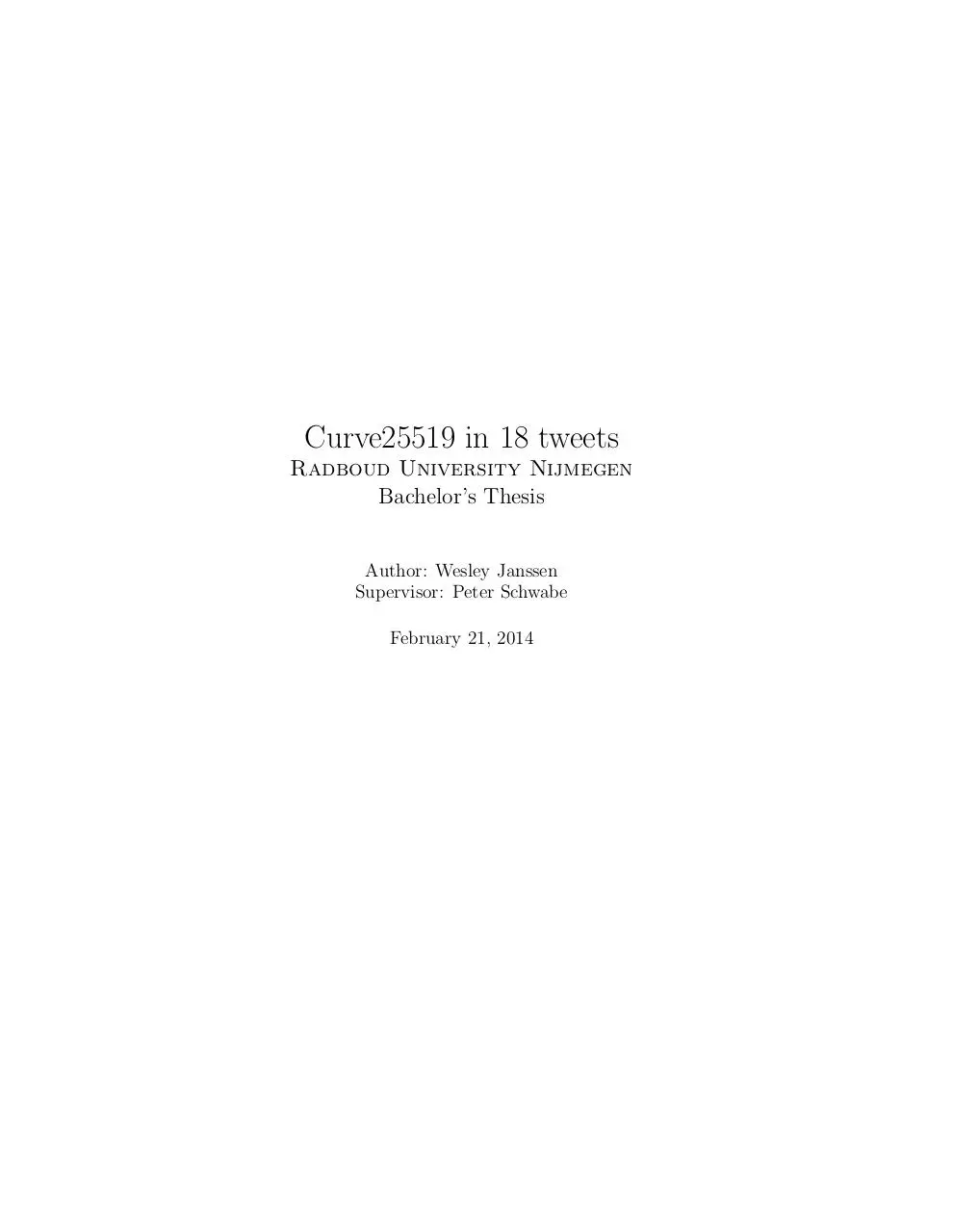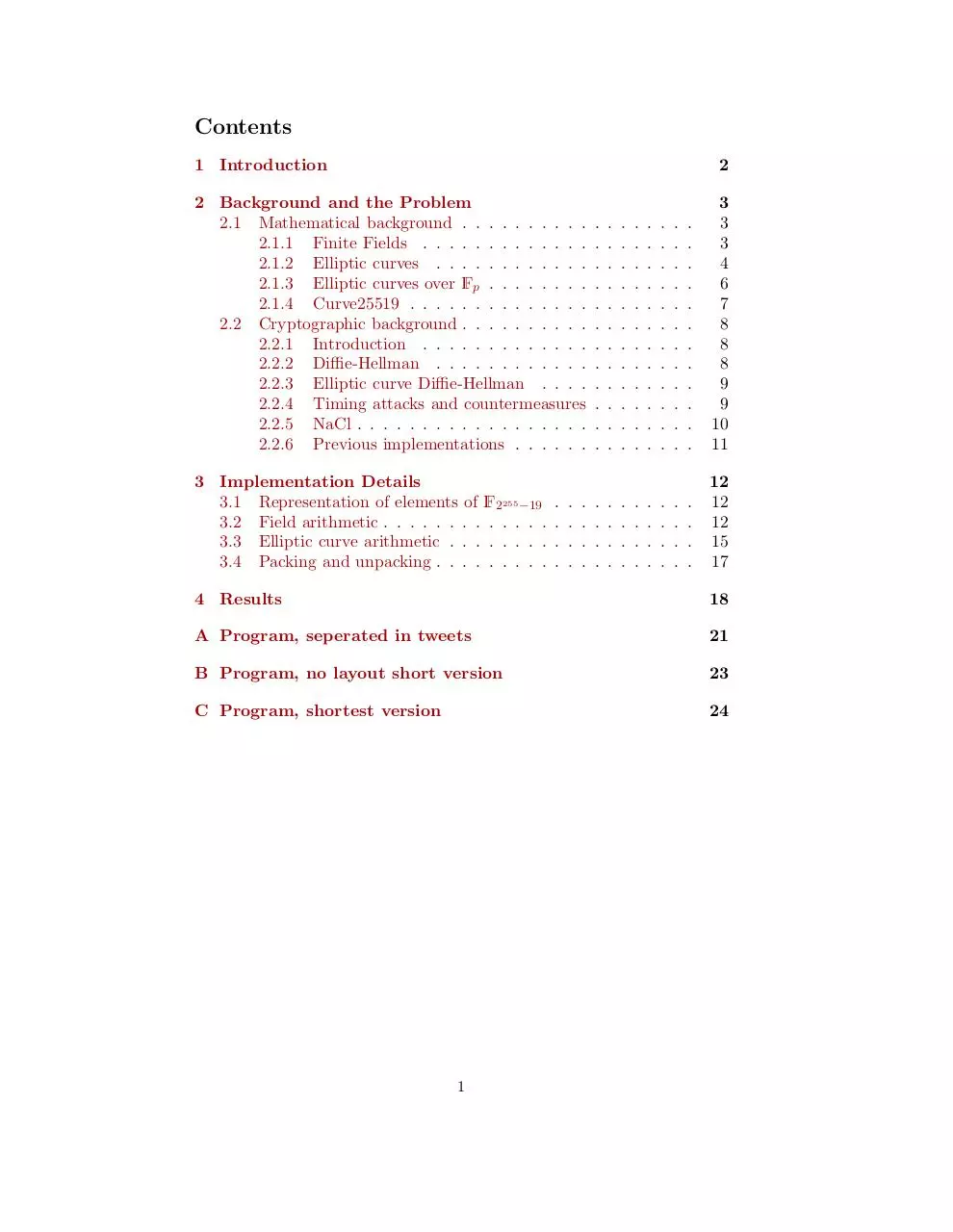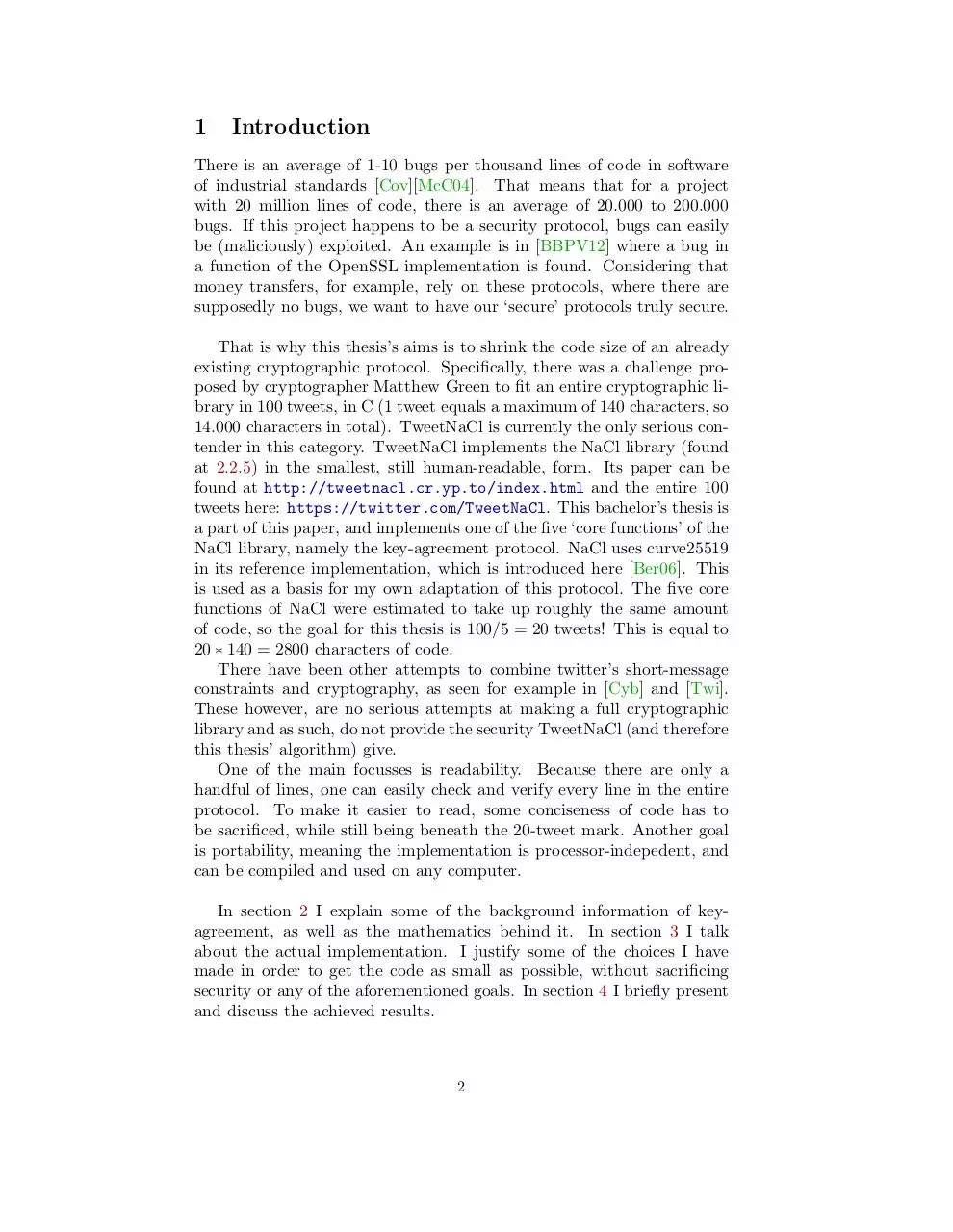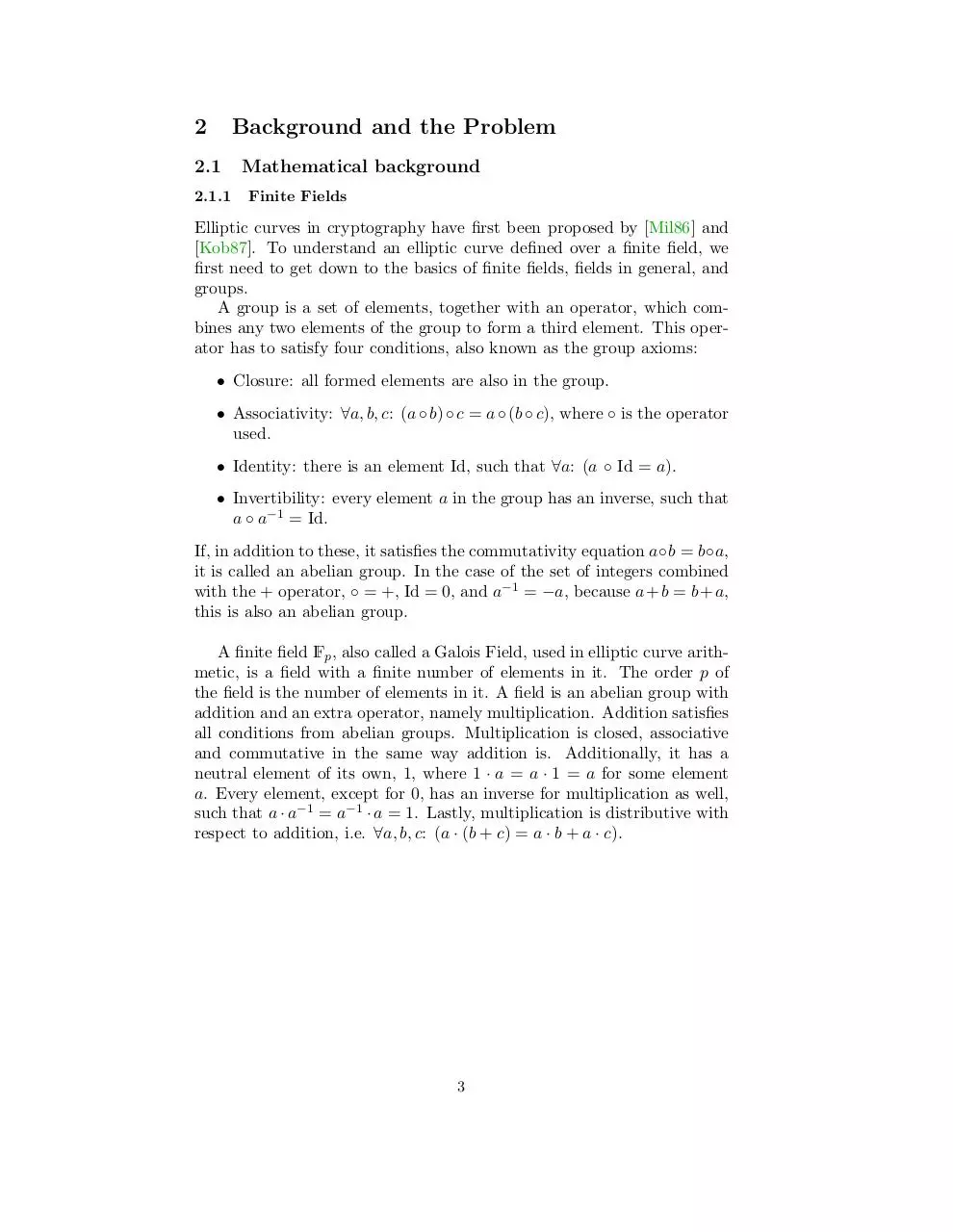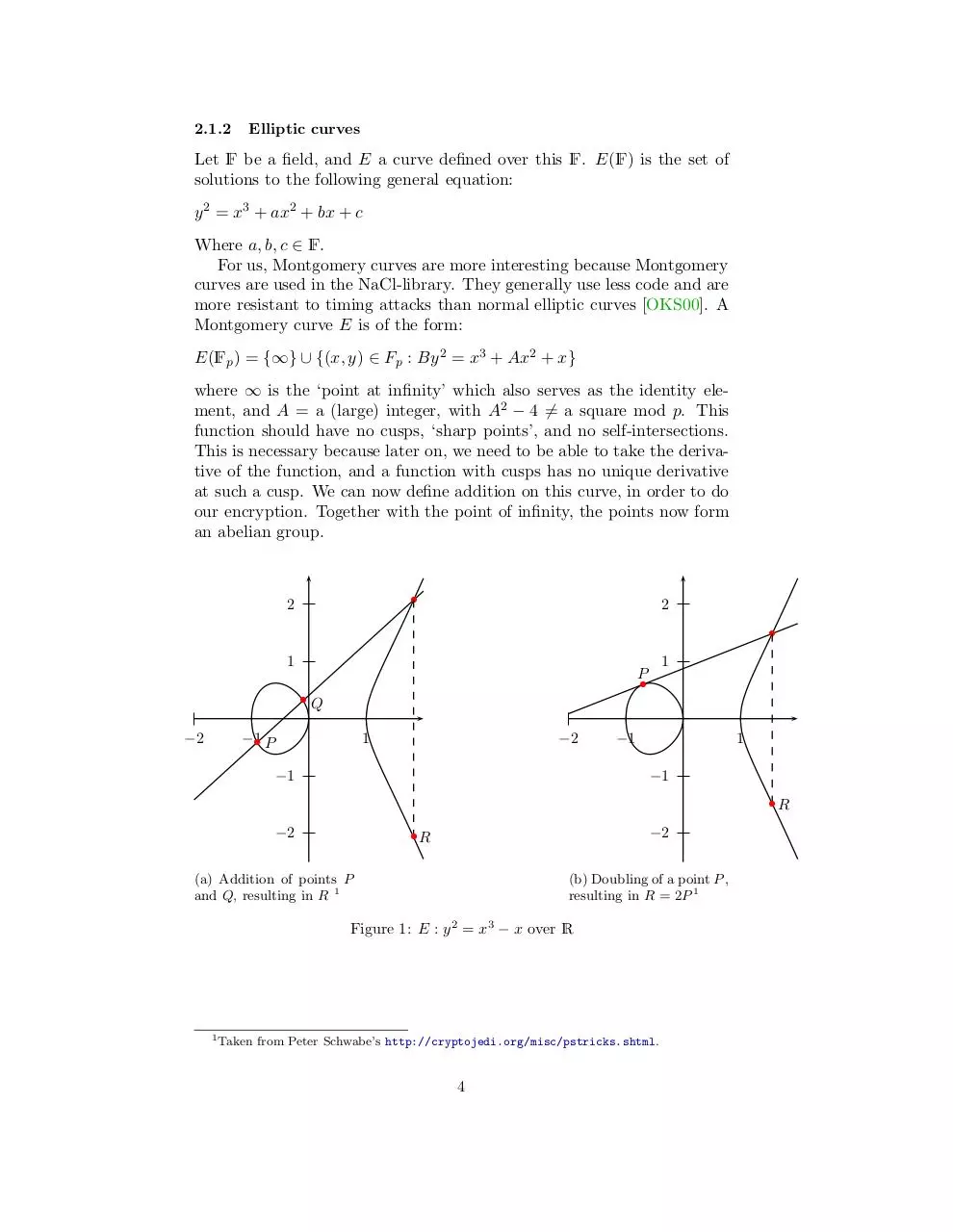BacTesWesley.pdf (PDF, 227.95 KB)

### Share on social networks

#### HTML Code

Copy the following HTML code to share your document on a Website or Blog